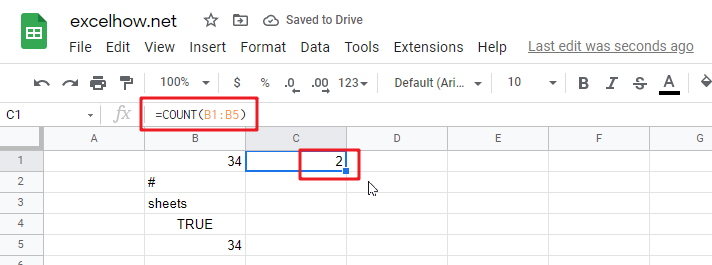# ExcelHow

This post will guide you how to use Google Sheets COUNT function with syntax and examples in Google Spreadsheets.

## Description

The Google Sheets COUNT function counts the number of cells that contain numbers, and counts numbers within the list of arguments. It returns a numeric value that indicate the number of cells that contain numbers in a range.

The COUNT function is a build-in function in Google Spreadsheets and it is categorized as a Statistical Function.

## Syntax

The syntax of the COUNT function is as below:

`= COUNT(value1, [value2],…)`

Where the COUNT function arguments are:

• `value1 `-This is a required argument.  The first item, cell reference, or range within which you want to count numbers.
• `Value2 `– This is an optional argument. You can enter up to 255 value arguments.

Note:

• Arguments that are numbers, dates, or a text representation of numbers (for example, a number enclosed in quotation marks, such as “1”) are counted.
• If the argument is an array or reference, only numbers in that array are counted. Empty cells, logical values, text, or error values in the array or reference are not counted.
• You can use the COUNTA function to count logical values, text, error values.
• You can use the COUNTIF function to count only numbers that meet certain criteria.

## Google Sheets COUNT Function Examples

The below examples will show you how to use Google Sheets COUNT Function to count the number of cells that contain numbers in a range.

#1 To count the number of cells in the range B1:B4, just using the following Google Sheets formula:

`=COUNT(B1:B4)`